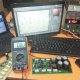Breaking News
Home / How to make an Amplifier? / how to make an amplifier

# how to make an amplifier

## how to make an amplifier?

Here in this article, we are going to know how to make an amplifier. In our technology have many kinds of an amplifier. Class A amplifier, Class B amplifier, Class AB amplifier, and Class D amplifier. There has an IC based amplifier. Transistor-based. we are going to learn about the transistor. In IC based amplifier is the fixed Watts amplifier. But transistor based are not. transistor based can be increased. We can make the amplifier according to our needs. how many Watts we need. Such as we need 500 Watts amplifier. for this cause, we have to calculate.

First, we need a 500 Watts transformer. We know that Voltage X ampere = Watts. If we use 50-0-50 Voltage. then we have to use the 10-ampere transformer. because voltage X ampere = Watts. So 50v X 10amp = 500 Watts. Now we have to calculate about the transistor. we know 1 transistor can take 1.2 amperes.  so we are using 50 voltage and 10 amperes. So 10/1.2=8.3 So we have to use a minimum of 8 transistors. But for the good performance, We use some more. like 12 transistor because 1 transistor can take maximum 1.2 amperes. Always we try to calculate 0.8 amperes per transistor. So 12X0.8=9.6 amperes. It will be better for the transistor. the transistor can’t be hot in load.

Here in this picture, we can see. we use a classic transformer and 2 pieces of transistor amplifier circuit board. here this circuit has 4 transistors. this transformer is 50-0-50 voltage and 6 amperes. this transformer is 50X6=300 Watts. S0 this is a 600 Watts amplifier. We use 2 100V 10,000uf Capacitor and 1 bridge diode. Please try to use the best quality diode.this is a 6-ampere transformer. we need the 10-ampere diode for this amplifier.

Now we are going to Watch the Video. how to make this amplifier.

transistor circuit diagram of 2sa1943 and 2sc5200

Thanks a lot to be with us. If you want another post then please visit our website.

We have another post for you. like as repairing amplifier,